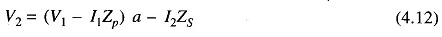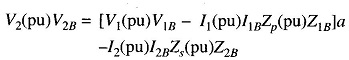## Per Unit System Definition:

Per Unit System Definition- It is usual to express voltage, current, voltamperes and impedance of an electrical circuit in per unit (or percentage) of base or reference values of these quantities. The Per Unit System Definition value of any quantity is defined as:The Per Unit System Definition method is particularly convenient in power systems as the various sections of a power system are connected through transformers and have different voltage levels.

Consider first a single-phase system. LetThenIf the actual impedance is Z (ohms), its Per Unit System Definition value is given byFor a power system, practical choice of base values are:In a three-phase system rather than obtaining the Per Unit System Definition values using per phase base quantities, the per unit values can be obtained directly by using three-phase base quantities. Let

Three-phase base megavoltamperes = (MVA)B

Line-to-line base kilovolts = (kV)B

Assuming star connection (equivalent star can always be found),When MVA base is changed from (MVA)B, to to (MVA)B, B, new, and kV base is changed from (KV)B,old  to (kV)B, new, the new per unit impedance from Eq. (4.9) is given by### Per Unit Representation of a Transformer

It has been said in Section 4.2 that a three-phase transformer forming part of a three-phase system can be represented by a single-phase transformer in obtaining per phase solution of the system. The delta connected winding of the transformer is replaced by an equivalent star so that the transformation ratio of the equivalent single-phase transformer is always the line-to-line voltage ratio of the three-phase transformer.

Figure 4.7a represents a single-phase transformer in terms of primary and secondary leakage reactances Zp and Zs and an ideal transformer of ratio 1 : a. The magnetizing impedance is neglected. Let us choose a voltampere base of (VA)B and voltage bases on the two sides of the transformer in the ratio of transformation, i.e.From Fig. 4.7a we can writeWe shall convert Eq. (4.12) into per unit formDividing by V2B throughout and using base relations (4.11 a, b, c), we getEquation (4.13) can therefore be written asEquation (4.14) can be represented by the simple equivalent circuit of Fig. 4.7b which does not require an ideal transformer. Considerable simplification has therefore been achieved by the Per Unit System Definition method with a common voltampere base and voltage bases on the two sides in the ratio of transformation.

Z(pu) can be determined directly from the equivalent impedance on primary secondary side of a transformer by using the appropriate impedance base.

On primary side:On secondary side:Thus the per unit impedance of a transformer is the same whether computed from primary or secondary side so long as the voltage bases on the two sides are in the ratio of transformation (equivalent per phase ratio of a three-phase transformer which is the same as the ratio of line-to-line voltage rating).

The pu transformer impedance of a three-phase transformer is conveniently obtained by direct use of three-phase MVA base and line-to-line kV base in relation (4.9). Any other impedance on either side of a transformer is converted to pu value just like Zp or Zs.

### Per Unit Impedance Diagram of a Power System

From a one-line diagram of a power system we can directly draw the impedance diagram by following the steps given below:

1. Choose an appropriate common MVA (or kVA) base for the system.
2. Consider the system to be divided into a number of sections by the Choose an appropriate kV base in one of the sections. Calculate kV bases of other sections in the ratio of transformation.
3. Calculate per unit values of voltages and impedances in each section and connect them up as per the topology of the one-line diagram. The result is the single-phase per unit impedance diagram.

The above steps are illustrated by the fallowing examples.